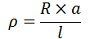# Difference Between Resistance & Resistivity

One of the major difference between the resistance and the resistivity of the material is that the resistance opposes the flow of free electrons whereas the resistivity is the property of the material which defines the resistance of the material having specific dimension. The other differences between them are explained below in the comparison chart.

## Comparison Chart

Basis For ComparisonResistance Resistivity
Definition Property of substance due to which it opposes the flow of electrons.It is defined as the resistance of material having specific dimensions.
FormulaSI Unit Ohms Ohms-meter
Symbol R ρ
Dependence Length, cross-section area of conductor and temperature. Temperature

## Definition of Resistance

The resistance is the property of the material which creates an obstruction in the flow of the current. When the voltage is applied across the conductor, the free electrons starts moving in a particular direction. While moving these electrons collapse with atoms or molecules and hence produce heat. These atoms or molecules oppose the movement of free electrons in a material.This opposition is known as the resistance. It is represented by the formulaWhere l – length of the conductor
a – cross section area of the conductor
ρ – resistivity of the material.

The SI unit of the material is ohms, and it is denoted by Ω at kΩ.

## Factors Affecting Resistance

The resistance of the wire depends on the following factors.

1. The resistance of the wire increases with the length of the conductor.
2. It is inversely proportional to the cross section area of the conductor.
3. It depends on the material of the wire.
4. The resistance of the material depends on their temperature.

## Definition of Resistivity

The resistivity is also known as specific resistance. The resistivity represents the resistance of the material which has specific dimensions, i.e., the material has 1-meter length and 1 square meter area of cross section.The formula represents the resistivity of the materialWhere l – length of the conductor
a – cross-section area of conductor
R – Resistance of the material

The SI unit of resistivity is ohm meter. The resistivity is directly proportional to the temperature of the material.The resistivity of the cube having one-meter side is defined as the resistance offered between the opposite two phases of the one-meter cube.

## Key Differences between Resistance and Resistivity

1. The resistance is the property of the material which obstructs the flow of current, whereas the resistivity gives the resistance of the material which has fixed dimension.
2. The resistance is the ratio of the length and cross-section area of the conductor, whereas the resistivity of the material is the ratio of the product of the resistance and area to the length of the conductor.
3. The resistance is represented by the symbol R whereas the resistivity is represented by the symbol ρ.
4. The SI unit of the resistance is ohm, and the SI unit of resistivity is ohm-meter.
5. The resistance of the material depends on the length, cross-section and area of conductor whereas the resistivity depends on the nature and temperature of the material.

The inverse of the resistivity is known as the conductivity of the material.